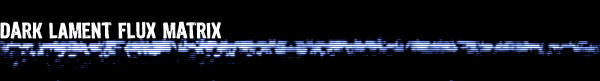written by Eldritch A Flux Matrix is a Dark Lament designed piece of Science Friction that looks like a small, purple liver. It is completely still when unloaded, but when loaded with preformulated Ebb calculations the Matrix pulses with low frequency. In order to function properly, a Flux Matrix must be grafted onto the user's DeathSuit - it fits tightly onto the wrists portion of the suit. To activate the Matrix the user places a hand over the Matrix and activates the desired calculation with a short Formulaic equation. A Flux Matrix is a sentient Ebb Organism that can store Ebb Calculations. As Ebb Calculations are dynamic, storing precalculated equations is not simple; too many variables can change from moment to moment. But the Flux Matrix can compute these variables indipendently; thus it is quasi a bionic DeathSuit co-processor. While the DeathSuit acts as calculation enhancer, the Flux Matrix can read ambient Flux variables, compute their values in prestored equations and quickly feed the complete equation into the user's brain, so that he can execute them without having to calculate them himself. There are a variety of Flux Matrixa, distinguished by the number of equations they can store. Standard Dark Lament Matrixa come in 3, 7, 9 and 13 equation Matrixa, costing 699, 999, 1333 and 3333 Credits respectively. To store an Ebb equation into the Flux Matrix, the user precalculates the Ability in a non-stressful situation. He rolls a standard CONC-test, to see if he correctly calculates the Ability and identifies all situational variables correctly. Then he rolls a Formulae roll and adds the Success Die from the CONC-test. If the result indicates a success, the Ability is stored in the Matrix and can be executed any time the user wishes. Executing an equation stored in a Flux Matrix counts a no Action, but executing two equations does count as an Action. Thus a maximum of two equations can be executed from a Flux Matrix per Phase. To store an Equation the Ebon pays the FLUX needed for the Ability plus an additional 7 FLUX for storage. Any point scored on the Success Die of the Formulae roll reduces the total by one point. Note: Rumors that Flux Matrixa possess enough sentience to "take over" the Ebon are wholly unsubstantiated. The Flux Matrix uses DNotice-system terminology. The procedure for standard SLA system is as follows: To store an Ebb equation into the Flux Matrix, the user precalculates the Ability in a non-stressful situation. He rolls a standard CONC-test, to see if he correctly calculates the Ability and identifies all situational variables correctly. Then he rolls a Formulae roll and adds any points in excess of 21 scored in the previous CONC-test. To store an Equation the Ebon pays the FLUX needed for the Ability plus an additional 7 FLUX for storage. Any point scored on the Formulae-test in excess of 21 reduces the total by one point (up to a maximum of 6 points).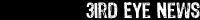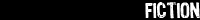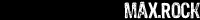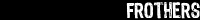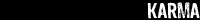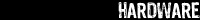In order to view the .pdf files from the Files Section you need the Acrobat Reader 4.0 or higher. Get if for free from Adobe.comVisit Nightfall Games Ltd.

 SLA Industries and all characters, settings, images and other intellectual properties pertaining thereto are (c) 1993-2000 Nightfall Games Limited, and are used without permission. No challenge to those copy-rights is intended or implied.

: website developed by eldritch/dnotice.de : tested on mozilla firefox 1.0 & internet explorer 6.0 : minimum resolution 1024x768 :